Roadbook Quick Start Quiz DIFFICULT VERSION (BETA TEST)
TESTERS: Please take the EASY VERSION QUIZ first...

Copy and paste this URL into your browser before you attempt the difficult version.
https://forms.gle/UPMDLBedUZRX6qPbA

***The quiz and the material it's based on are in the development stage. You feedback is appreciated.
IMPORTANT NOTE FOR BETA TEST TAKERS: Write in answers in ALL CAPS. If your "write in" answer is numeric, use a decimal point (not a comma) and do NOT add KM or KMS after the number.

demonstrates the most basic concepts of what comprises a roadbook, the information contained in a basic roadbook, the way the information is organized and how to read a basic roadbook. Some of the questions you will answer don't relate to rally navigation in the practical sense. Instead, these questions demonstrate you understanding of the information in a roadbook and how that information in organized.
Email *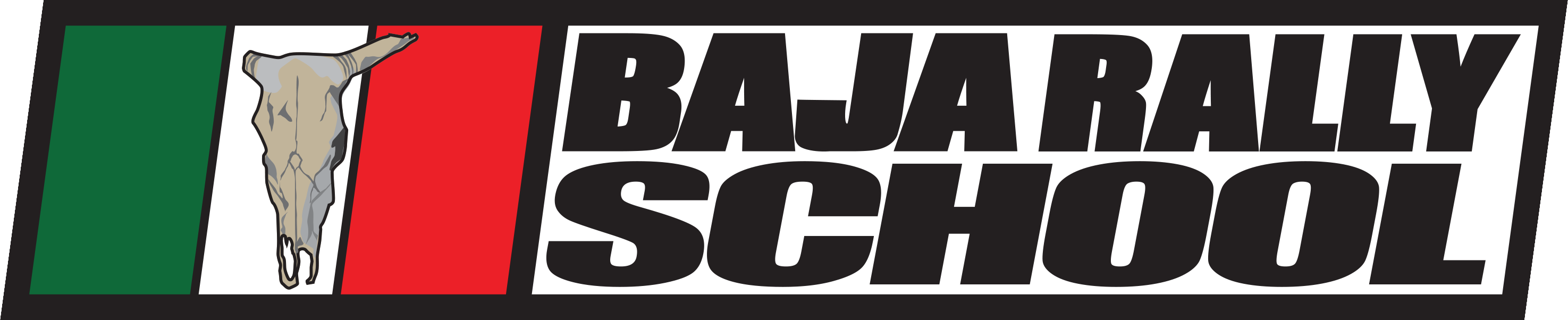Using the image below: Which note below describes the following event: KEEP STRAIGHT THROUGH THE INTERSECTION ALONG THE LEFT SIDE OF SAND WASH HEADING 034 DEGREES *
10 points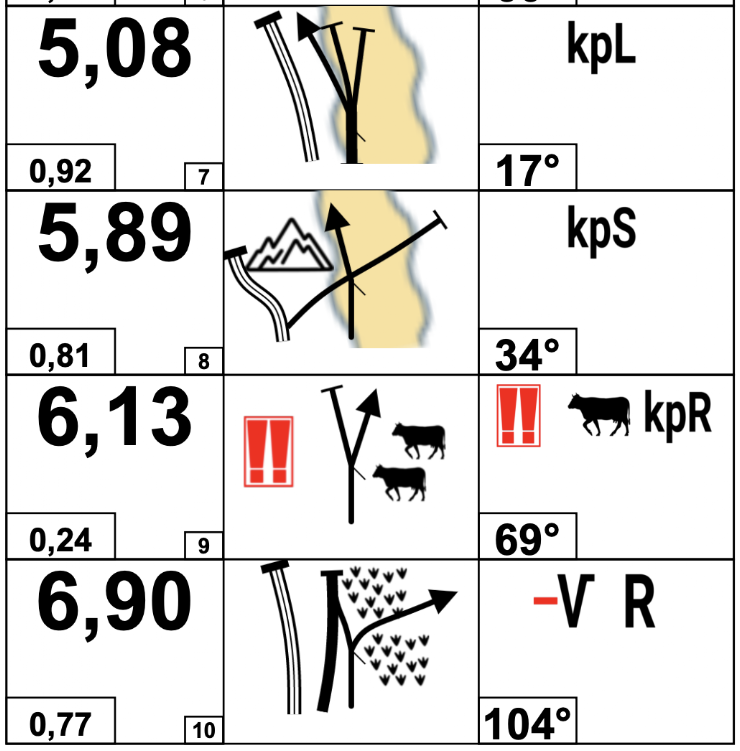What is the exact distance between Note #3 and Note #6? Your answer should be expressed in numeric value only in hundredths of kilometers (ie: 0.82 or 3.07) *
10 points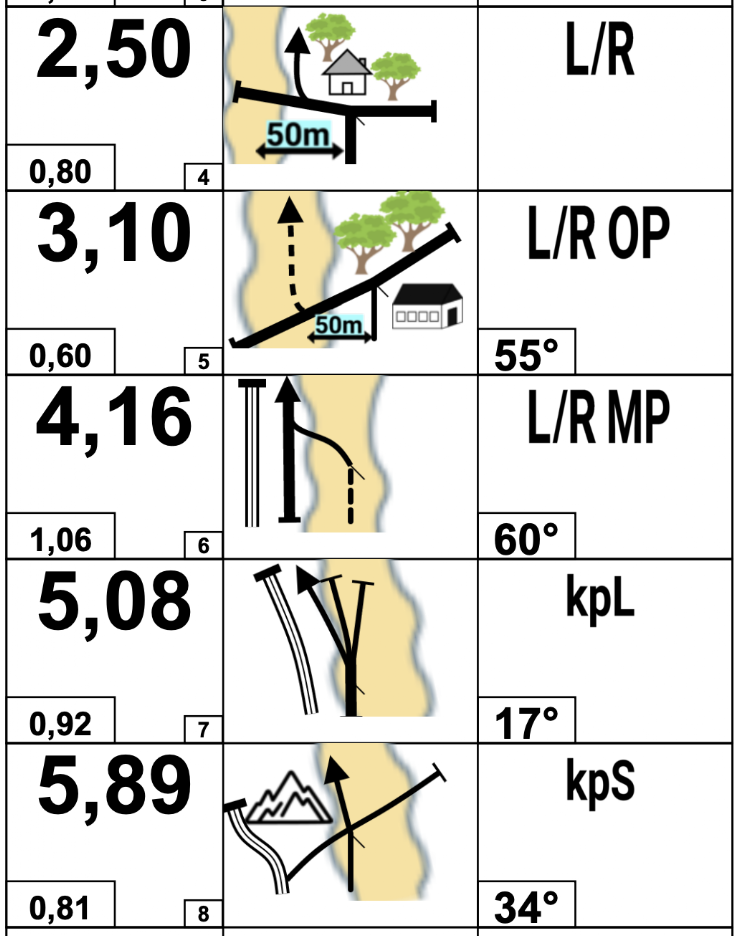How do you read the Left Box Diagram *
10 points
Which of the following is not some type of "event" *
10 points
Words, abbreviations & symbols describing an "event" are found in which box of a notation? *
10 points
10 points
Required
What is the most important number to know about Note #121 below: *
10 points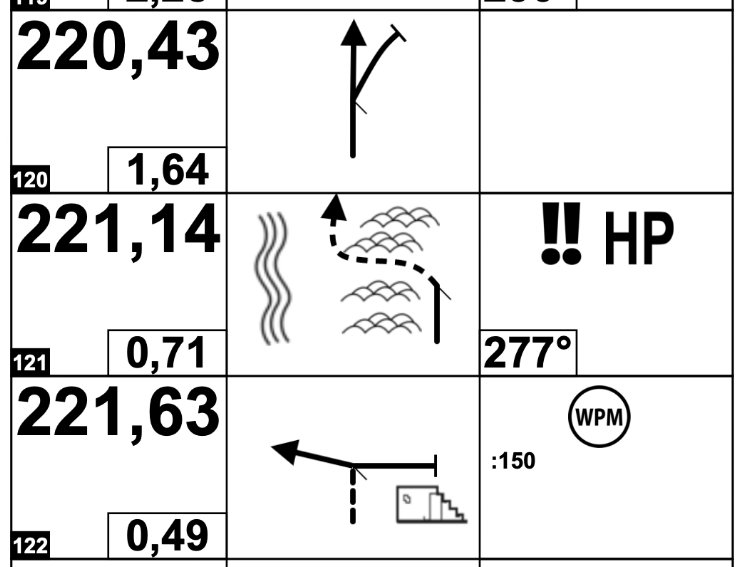What is the approximate distance between the left and right turn in Note #4 below *
10 points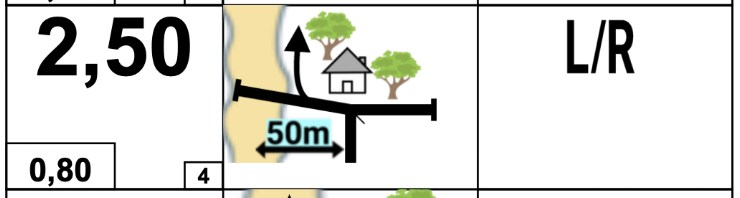The Center Box should be read: *
10 points
What is the specific safety hazard to be aware of in this section of route below? WRITE YOUR ANSWER IN ONE WORD ONLY, ALL CAPITAL LETTERS *
10 points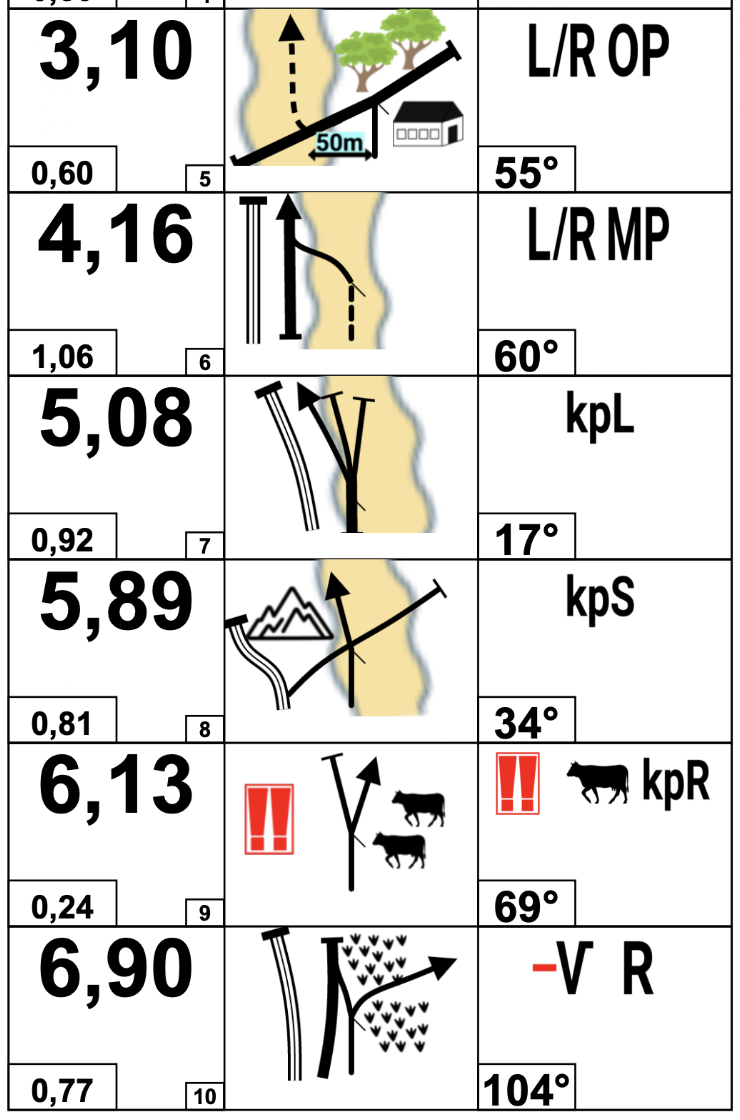Using the same section of roadbook from the question above, at what distance from the previous note does the safety hazard occur? Your answer should be expressed in numeric value only in hundredths of kilometers (ie: 0.82 or 3.07) *
10 pointsWhat is the exact distance in meters from the beginning of the 2nd dune section to the triple hazard? *
10 points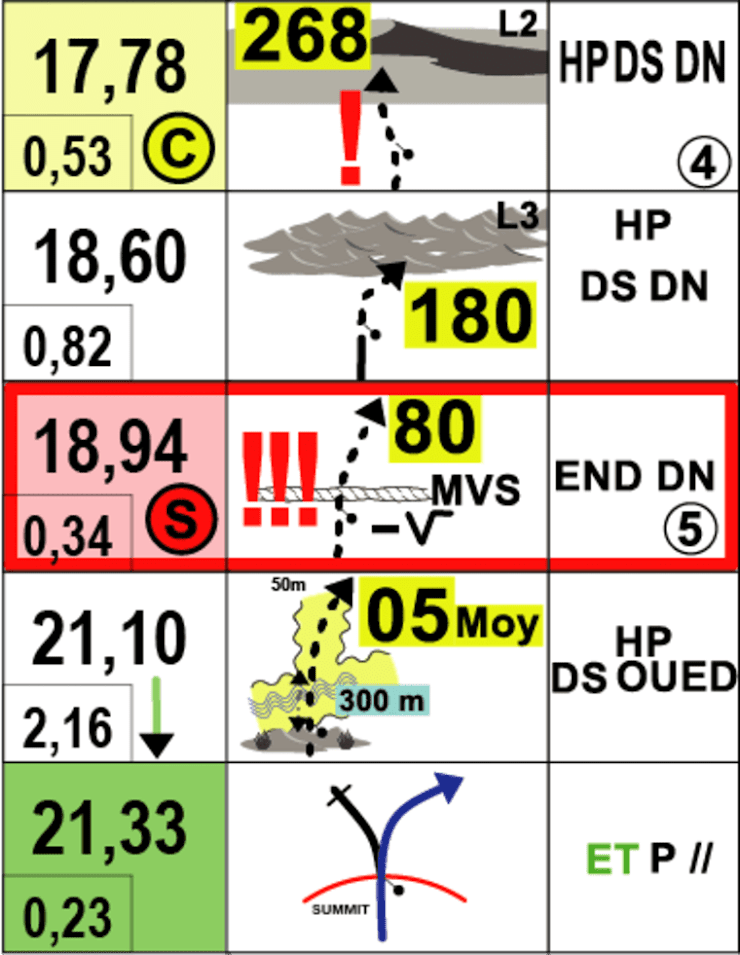In what box will you always find a compass heading on a note in the roadbook? *
10 points
From what perspective is the Center Box illustrated? *
10 points
In most cases except long sections of HP (off track navigating), if you had to navigate without any one of the 3 boxes in a roadbook notation which would it be? *
10 points
What is the exact distance from the prior note that you'll need to fork left for 0.10 KM before crossing another track Your answer should be expressed in numeric value only in hundredths of kilometers (ie: 0.82 or 3.07)
0 points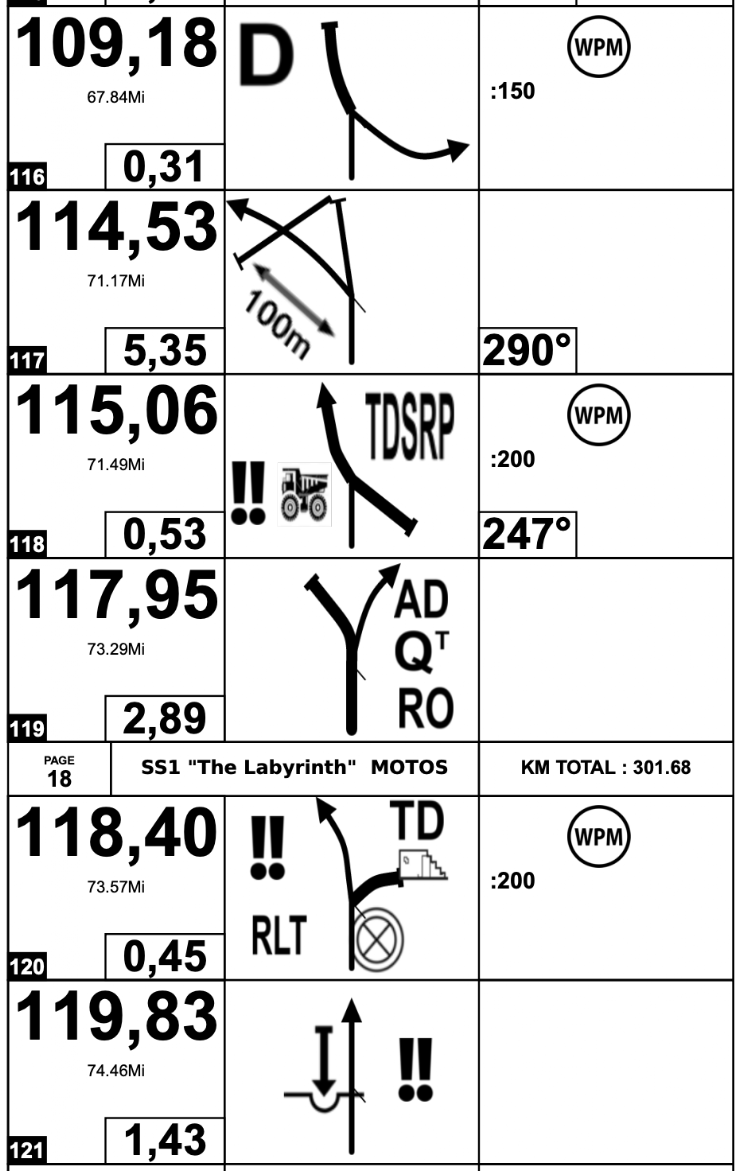What note number is explained by "Fork right and leave the main road"? *
10 pointsUsing the section of roadbook below, what is the exact mileage (Distance) of the first fixed hazard event that the hazard will always be present? Your answer should be expressed in numeric value only in hundredths of kilometers (ie: 0.82 or 3.07) *
10 points